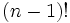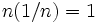Expected number of fixed points of permutation equals one

Suppose$n$ is a natural number. Consider the uniform distribution on the symmetric group of degree$n$, i.e., the symmetric group on a set of size$n$. The expected number of fixed points for a permutation picked according to the uniform probability distribution equals$1$.
We note that the number of permutations that fix a particular element$i$ in the set is$(n - 1)!$, hence the probability that$i$ is fixed is$1/n$. By linearity of expectation, the expected number of fixed points is the sum, for each point, of the probability that it is fixed. This sum is$n(1/n) = 1$.